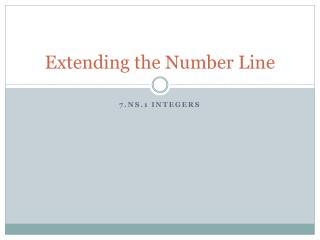DownloadDownload PresentationExtending the Number Line

# Extending the Number Line

Télécharger la présentation## Extending the Number Line

- - - - - - - - - - - - - - - - - - - - - - - - - - - E N D - - - - - - - - - - - - - - - - - - - - - - - - - - -
##### Presentation Transcript

1. Extending the Number Line 7.NS.1 Integers

2. Hot and Cold Running Weather Libya has some of the hottest weather on earth, with temperatures up to 136 °F. The coldest temperature recorded is at Vostok Station, Antarctica; a freezing −128 °F.

3. So what is the difference between them? Normal thermometers, like number lines, start at zero and go up, so the high temperature is easy to see. But negative temperatures are below zero so we need to look at a different type of number line.

4. Number lines can go both directions from zero −128 °F 136 °F These negative whole units, part of a group of numbers called integers, allow us to measure not only temperatures below zero but also debt, margins of error, population changes and a lot more.

5. What are Integers? Integers are all negative and positive whole units. They include all the natural and whole numbers but also include negative whole numbers. Integers …. -5, -4, -3, -2, -1, 0, 1, 2, 3, 4, 5….. Whole Numbers 0, 1, 2, 3, 4, 5…… Natural Numbers 1, 2, 3, 4, 5…

6. So what is the difference in the temperatures? −128 °F 136 °F 136 units from zero 128 units from zero So they are 264 units away from each other

7. There are a couple of important things to consider when comparing integers. A positive number is ALWAYS greater than a negative number. The more positive a number, the greater it is. Let’s look at an example. Example: -6 ____ 2 Think about the number line. Negative six is below zero. Two is above zero. Two is greater than negative six. -6 < 2

8. A real world way to think about it: In the real world we use negative numbers most when dealing with money. Positive numbers represent the money you have. Negative numbers represent the money you owe. When comparing -6 to 2, I know I would rather have two dollars than owe 6 dollars, so I know 2 is greater than -6. -6 < 2

9. Let’s try another -25 ____ -36 -25 means I owe 25 units. -36 means I owe 36 units. If my units were dollars, I would much rather owe only 25 dollars instead of owing 36 dollars. So I know that -25 is greater than -36. -25 > -36 Or I can still just think about how -36 is colder than -25.

10. Complete the Writing and comparing Integers worksheet. Then take the Quiz. Don’t forget to upload a copy of the worksheet to your teacher! Assignments